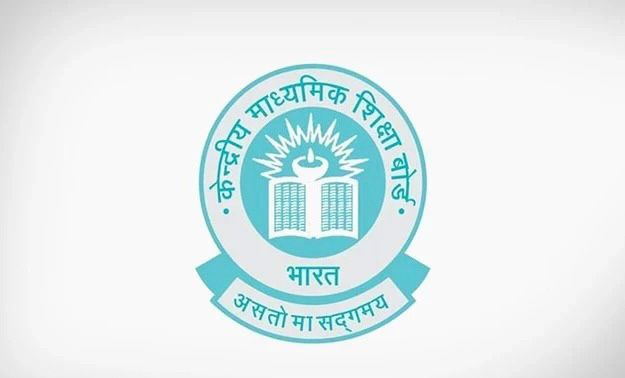# Two lines are given to be parallel. The equation of one of the lines is 3x –2y = 5. The equation of the second line can be

CBSE Maths Exam 2021-22 Term 1, question: Two lines are given to be parallel. The equation of one of the lines is 3x –2y = 5. The equation of the second line can bea) 9x + 8y = 7

b) -12x– 8y = 7

c) -12x + 8y = 7

d) 12x + 8y = 7